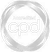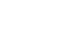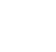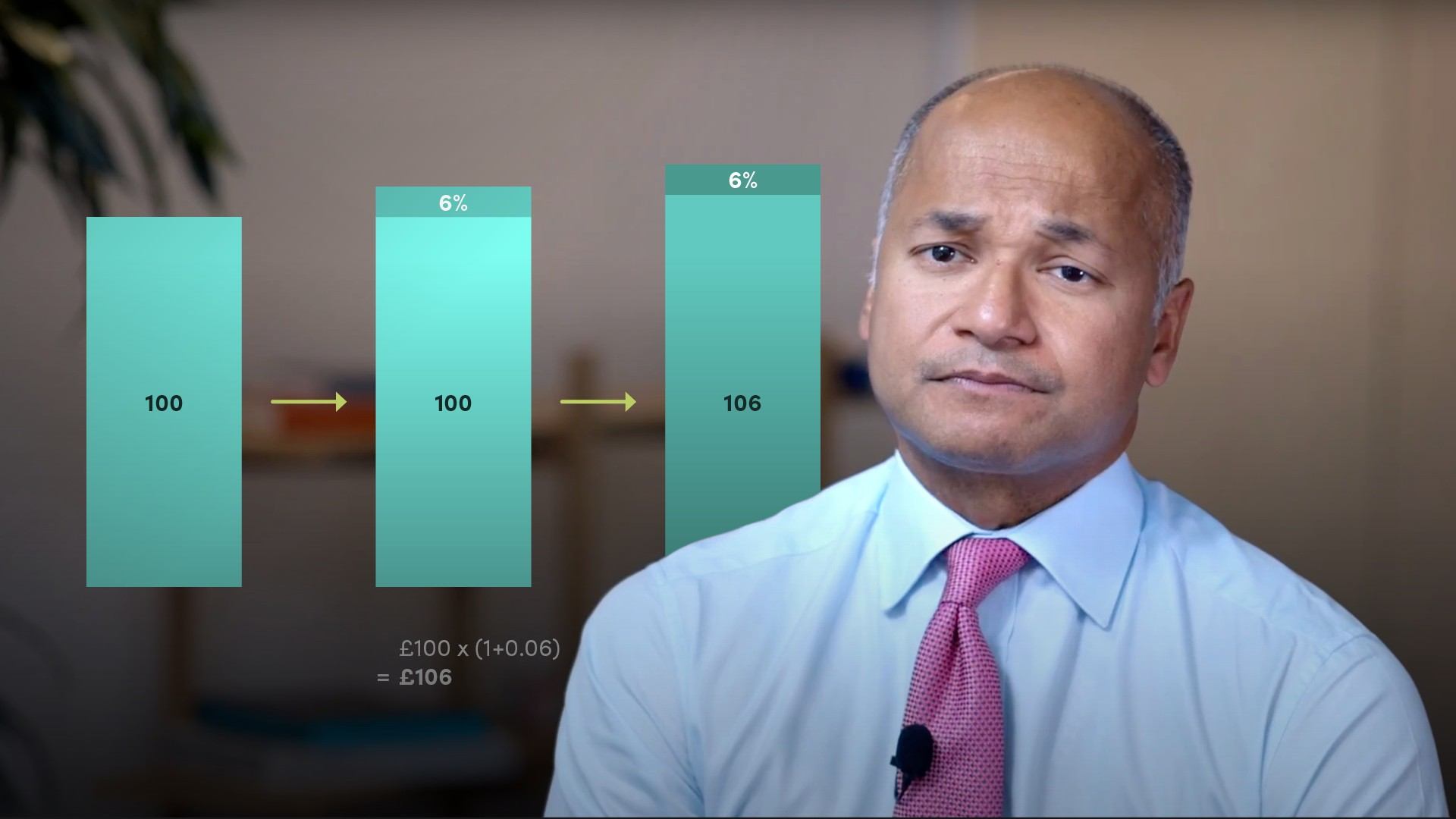# Introduction to Time Value of Money25 years: Treasury & ALM

Moorad begins his introduction to the Time Value of Money by discussing interest rate conventions and calculations.

Financial MathsMoorad begins his introduction to the Time Value of Money by discussing interest rate conventions and calculations.

Finance Unlocked is the video learning platform built for finance professionals.### Introduction to Time Value of Money

9 mins 47 secs

Overview

The time value of money is an essential concept in financial markets. The idea that money is worth more in the future requires an understanding of future value and compounding interest rates over different terms.

Key learning objectives:

• Define the time value of money

• Understand how to calculate compound interest

## Book a demo to access

Summary

#### What is the Time Value of Money?

The principles of corporate finance, and of financial arithmetic, have long been used to illustrate the fact that £1 received today is not the same as £1 received at some time in the future. Faced with a choice between receiving £1 today or £1 in one year’s time, we would not be indifferent, because while money received today has value, it will more likely than not have greater value in the future - provided we were to receive interest on money that was not received today but deposited for receipt at a later date. This is the concept of time value of money, a fact of life that means that the promise of a receipt of funds in the future is acceptable, provided we are compensated for not receiving it today. And we quantify the time value of money by quoting a rate of interest on the money in question.

#### What is future value and simple interest?

A loan that has one interest payment on maturity is accruing simple interest. On short-term instruments, there is usually only one interest payment on maturity, hence simple interest is received when the instrument expires. The terminal value of an investment with simple interest is given here:

• F = P(1+r)
• If P is £100, r is 5% and the investment term is one year then
• F = 100(1 + 0.05)
• = £105

The market convention is to quote interest rates as annualised interest rates, which is the interest that is earned if the investment term is one year. Consider a three-month deposit of £100 in a bank, placed at a rate of interest of 6%. In such an example the bank deposit will earn 6% interest for a period of 90 days. As the annual interest gain would be £6, the investor will expect to receive a proportion of this, which is calculated as follows:

• £6 * 90/365

This equals a receipt of £1.479 interest at the end of the 90-day term. The total proceeds after the three months is therefore, £100 plus £1.479. If we wish to calculate the terminal value of a short-term investment that is accruing simple interest we use the following expression:

• F = P ( 1 + r * (DAYS/YEARS) )

#### What is compound interest?

Let us now consider an investment of £100 made for three years, again at a rate of 6%, but this time fixed for three years. At the end of the first year, the investor will be credited with interest of £6. Therefore, for the second year the interest rate of 6% will be accruing on a principal sum of £106, which means that at the end of year 2, the interest credited will be £6.36. This illustrates how compounding works, which is the principle of earning interest on interest.

What will the terminal value of our £100 three-year investment be?

In compounding, we are seeking to find a future value given a present value, a time period and an interest rate. If £100 is invested today (at time t0) at 6%, then one year later (time t1) the investor will have £100 x (1 + 0.6) = £106. In our example, both the original deposit capital plus the interest received is left in for another year, so at the end of year 2 (t2) we will have the following;

• £106 x (1 + 0.6)
• £100 x (1 + 0.6) x (1 + 0.6)
• = £112.36

#### How do we calculate compound interest for more than one year?

In general, if compounding takes place m times per year, then at the end of n years, m*n interest payments will have been made and the future value of the principal is given here:

•  FV = PV (1 + r/m)^mn

## Book a demo to access

ExpertFormer senior banker with almost 30 years experience. Moorad has held senior roles in commerical, investment and retail banking. He has also spent the last five years as a visiting professor.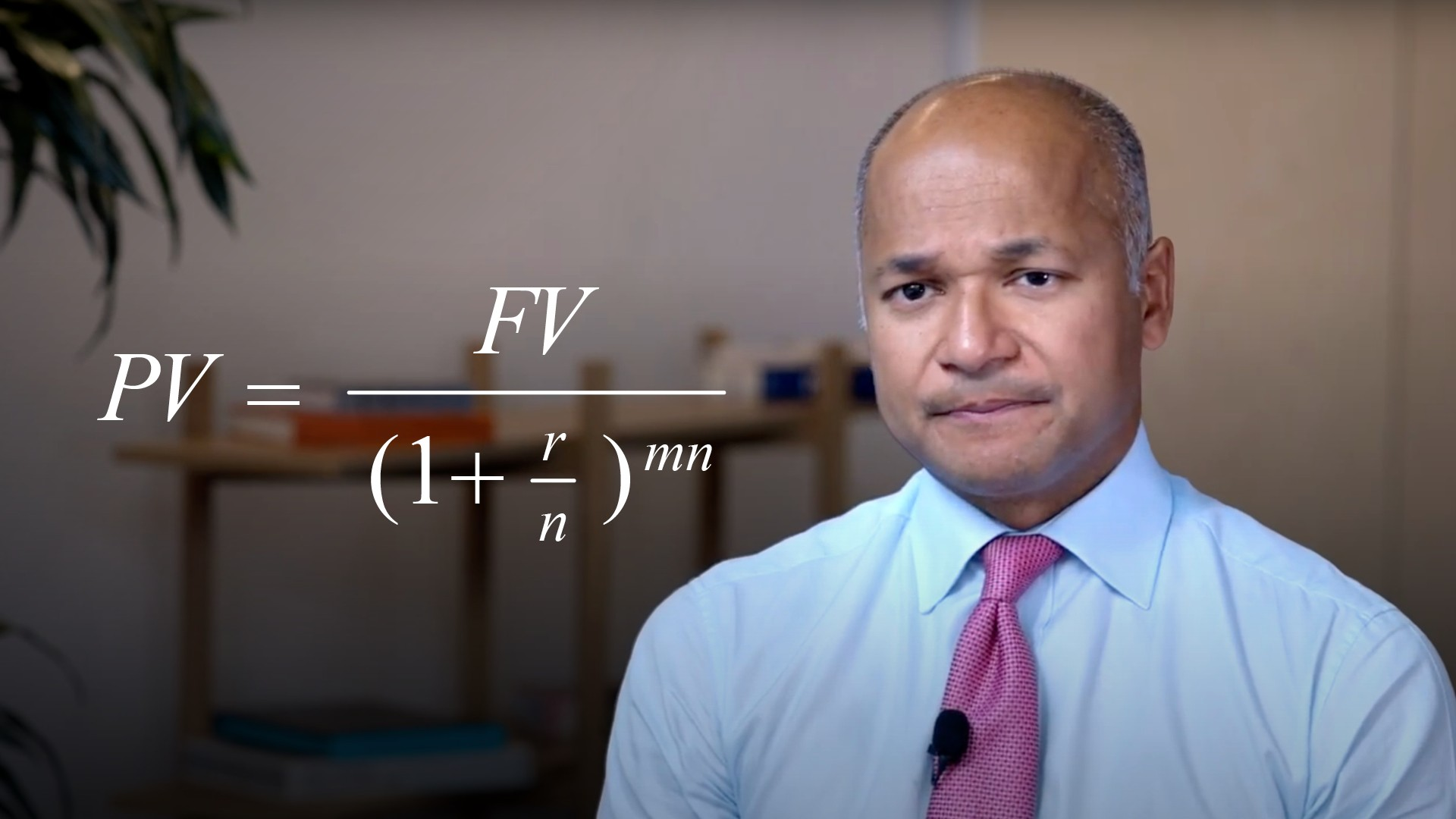09:58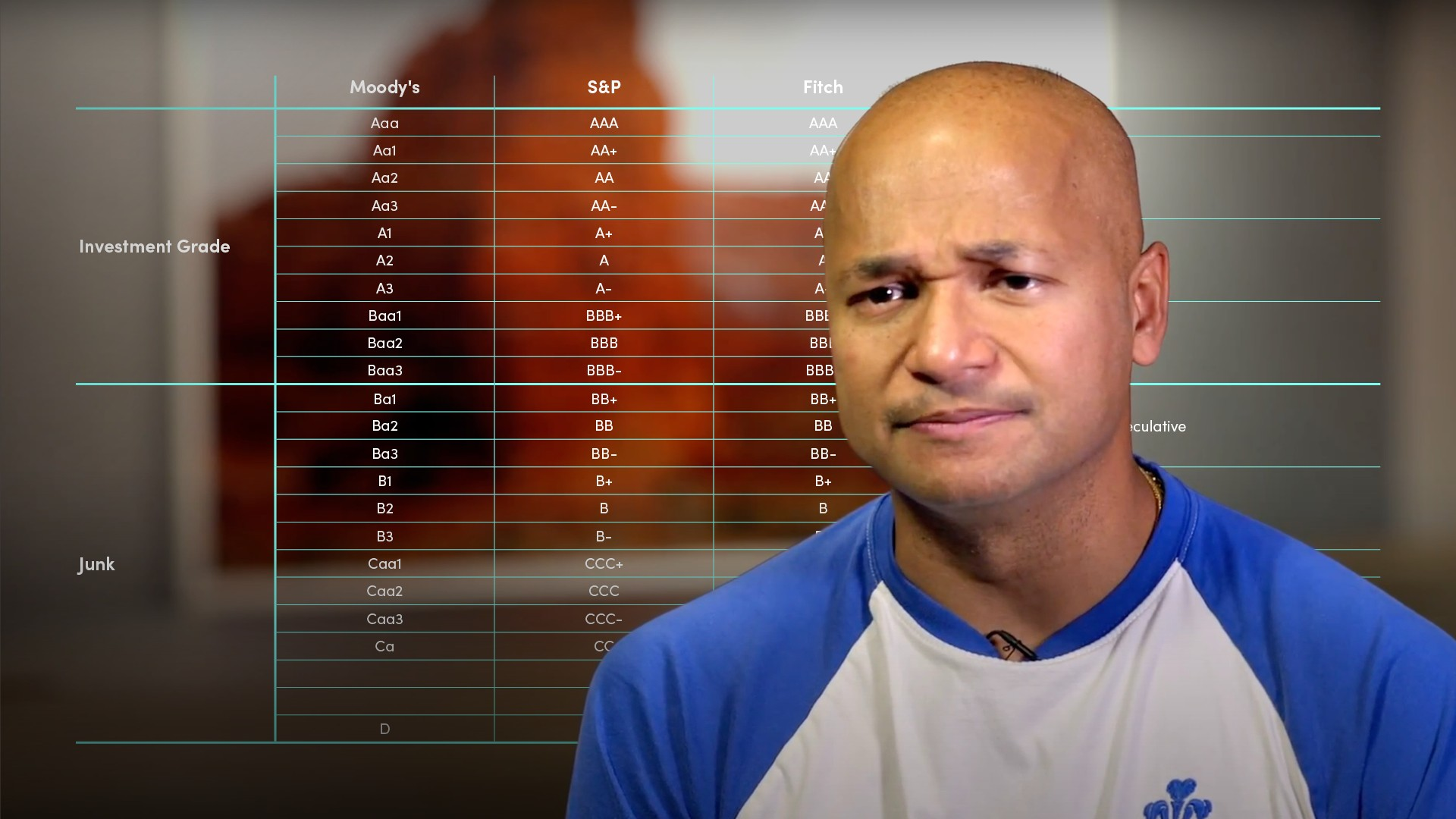06:2605:22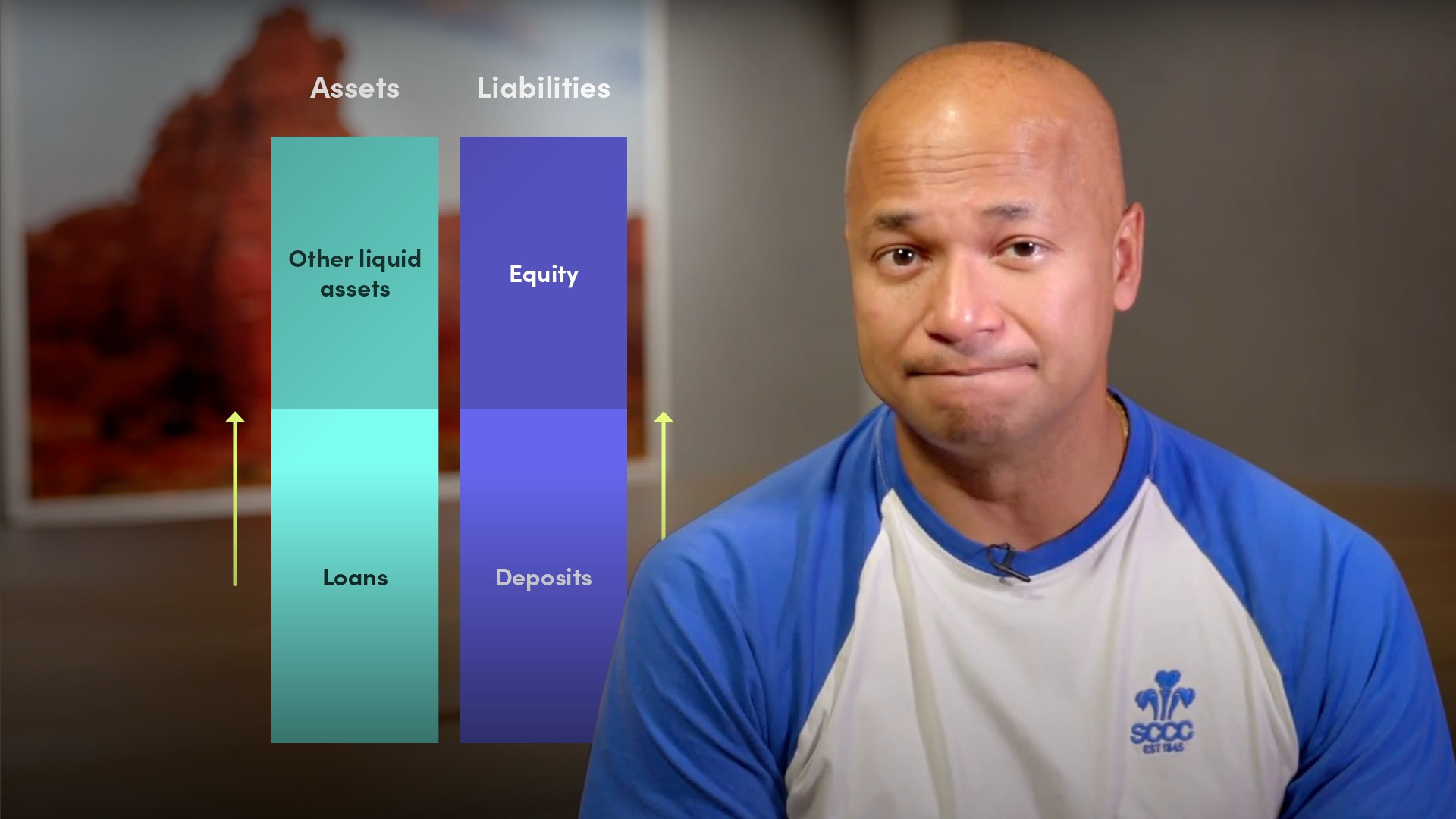03:26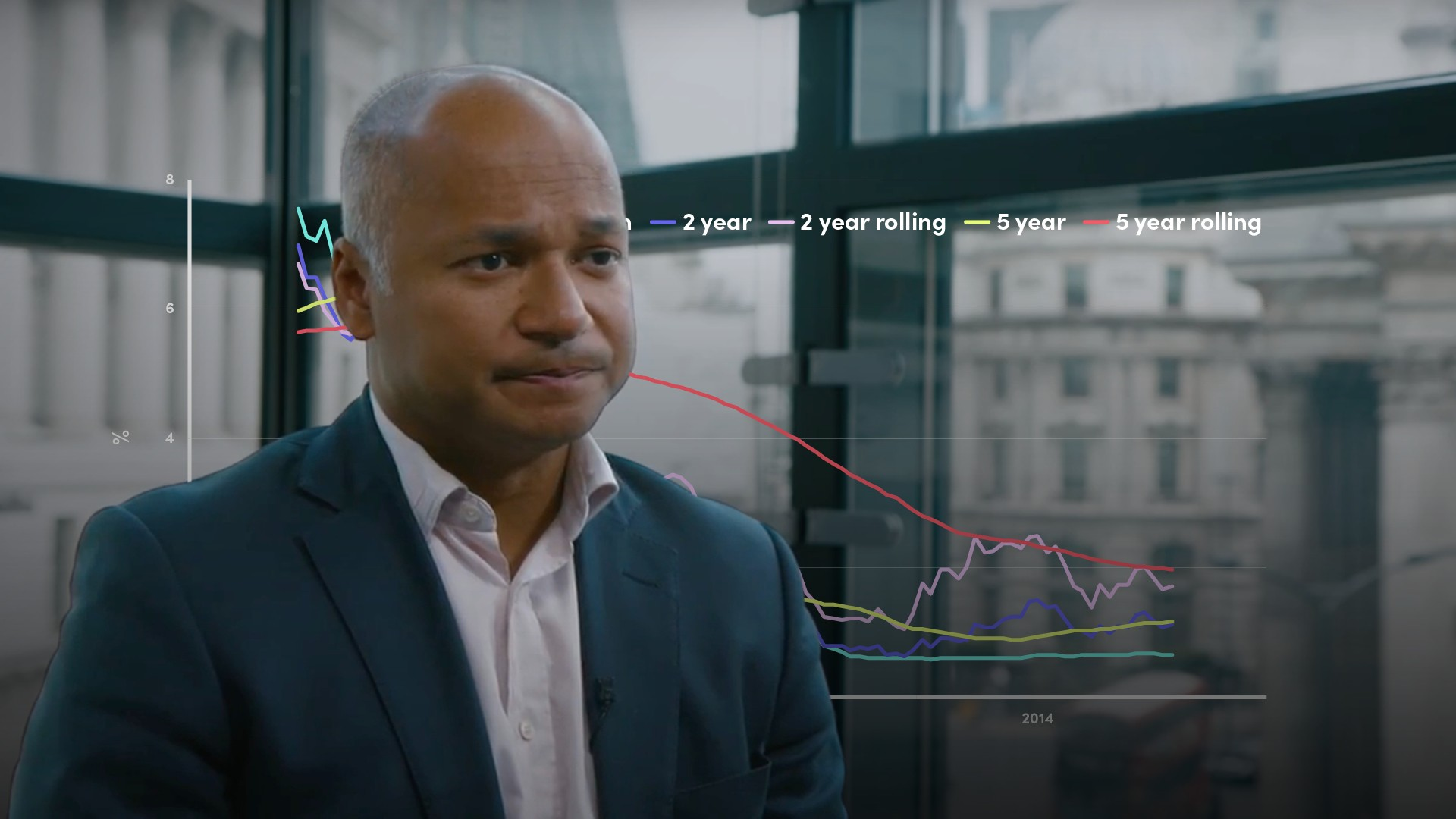14:5311:28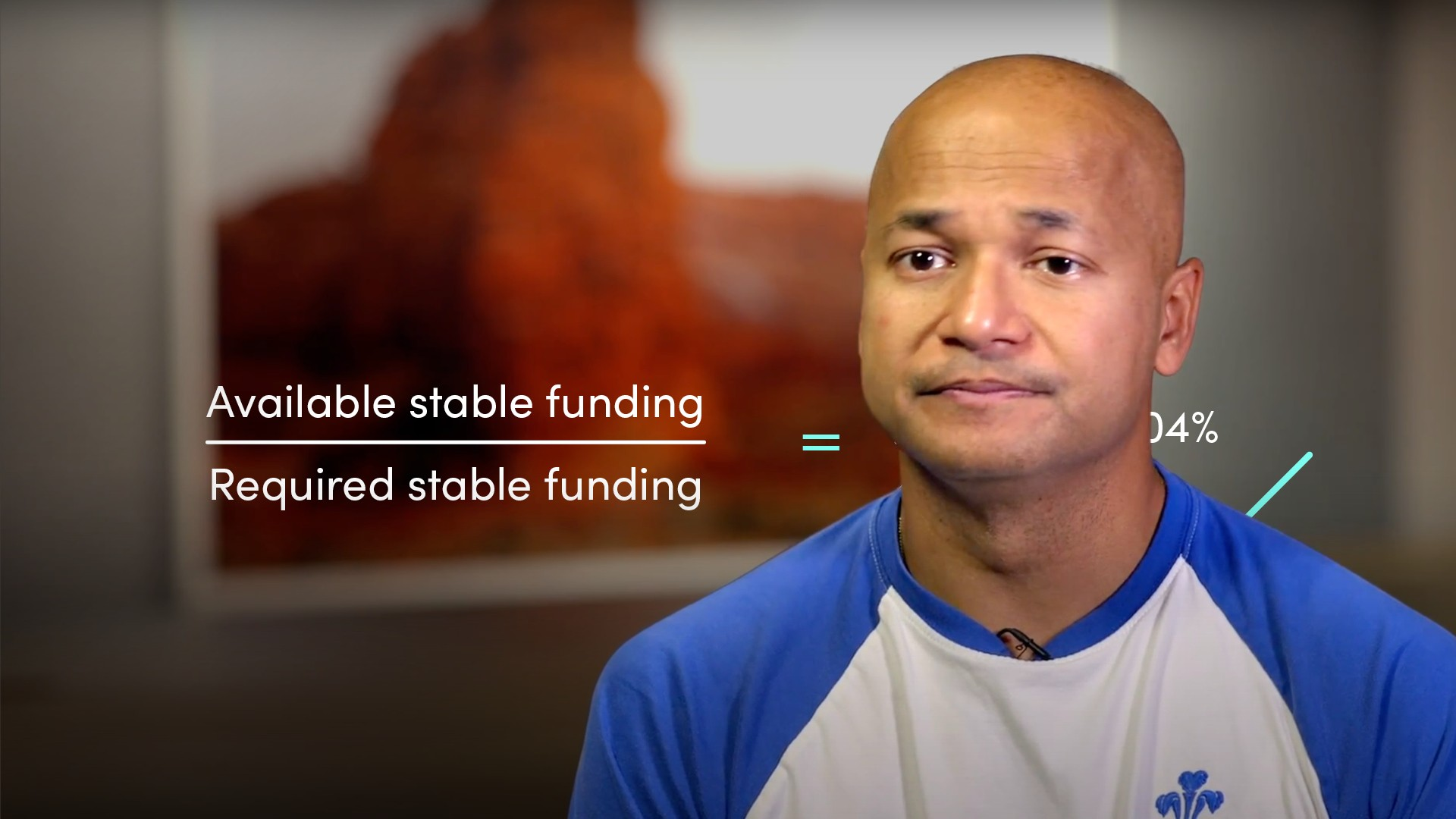07:35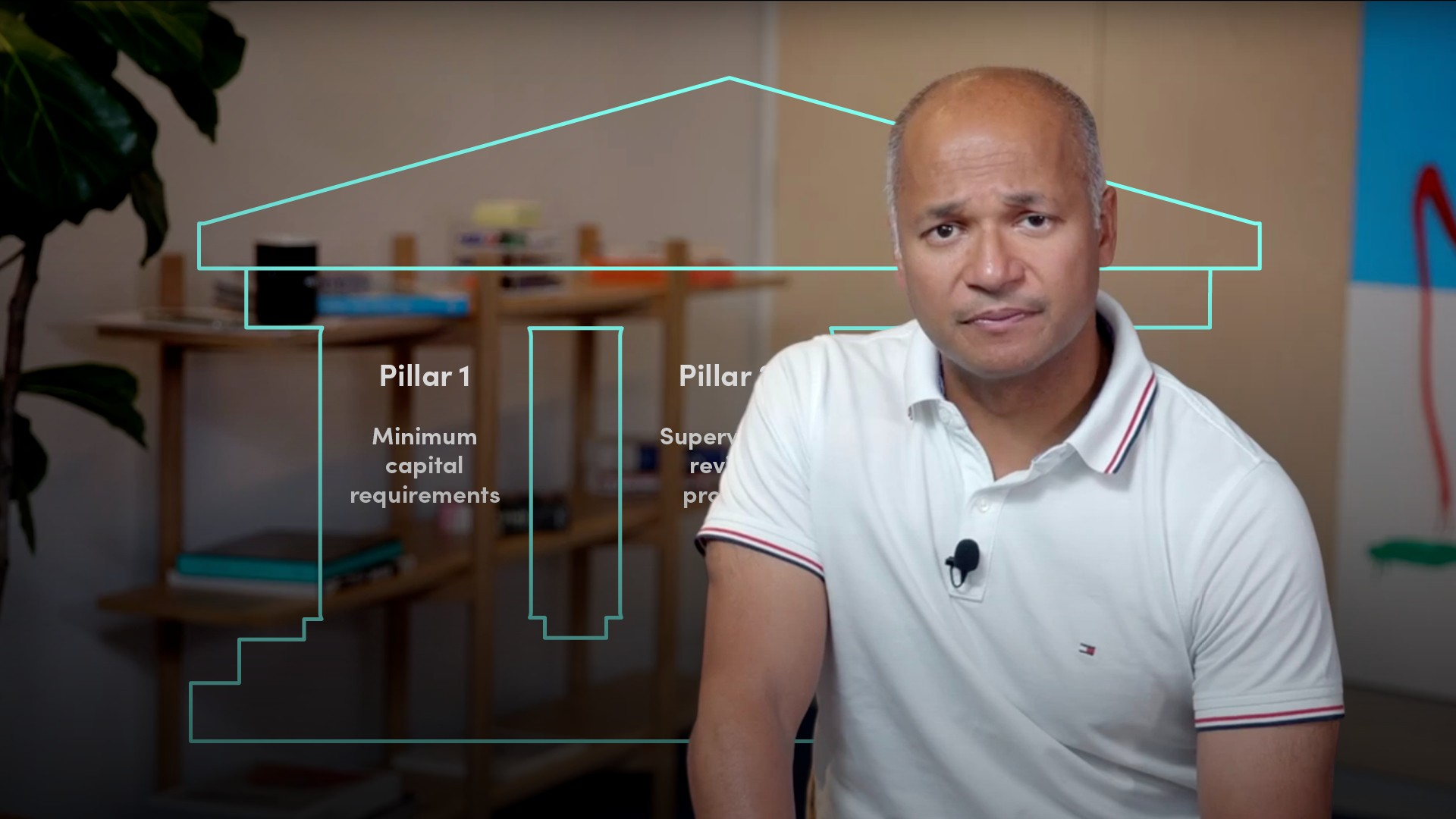13:19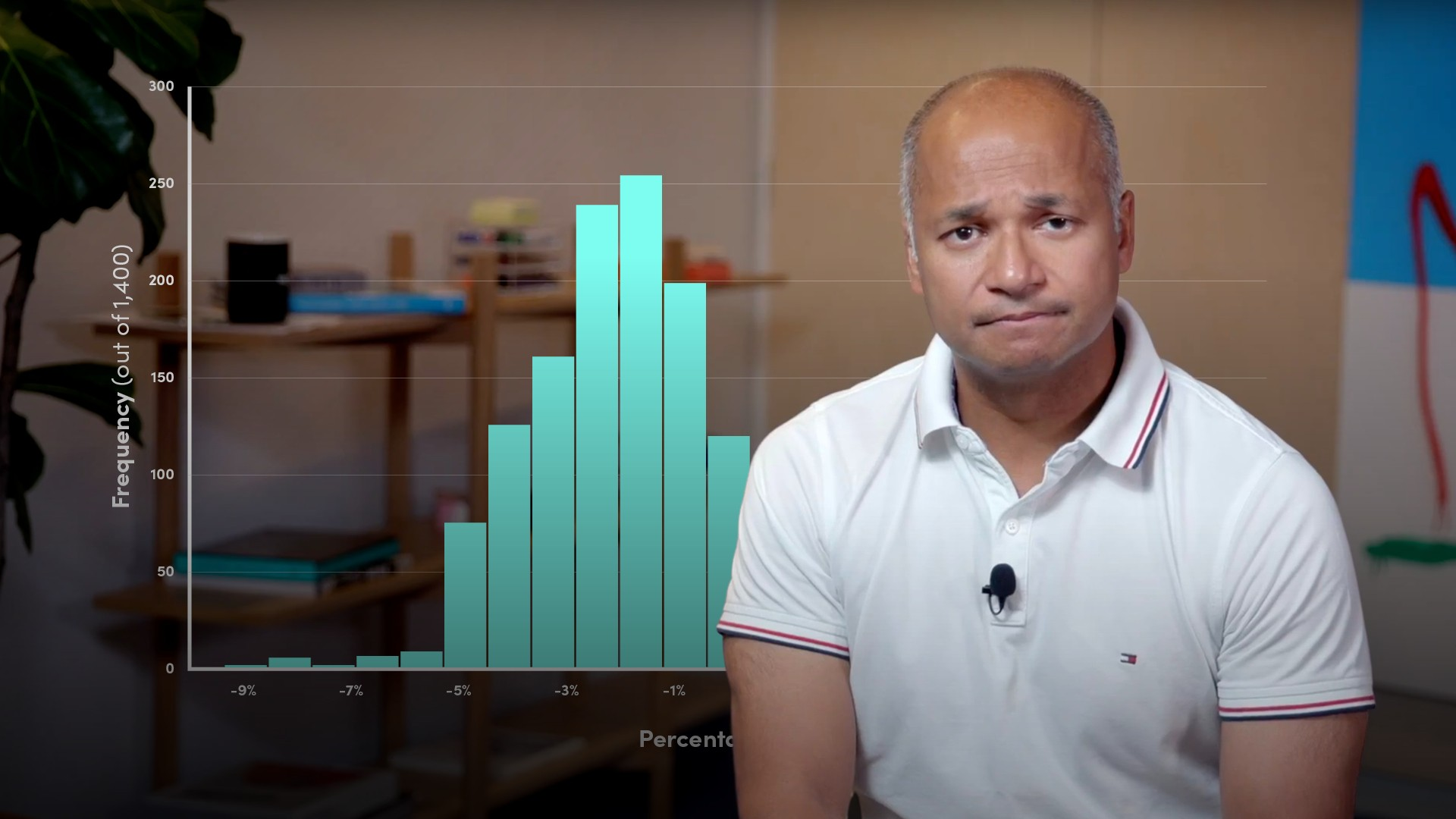15:25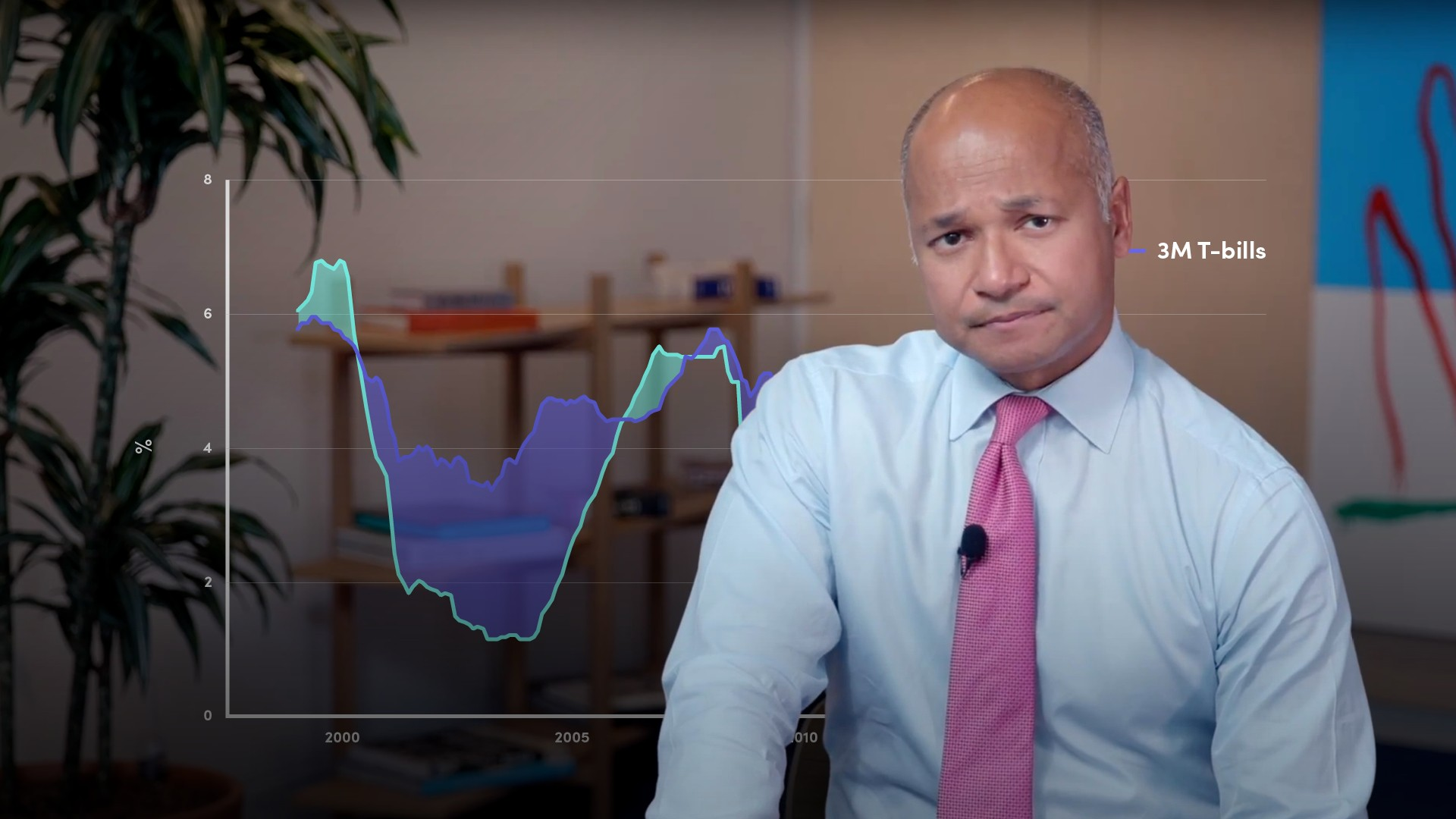11:47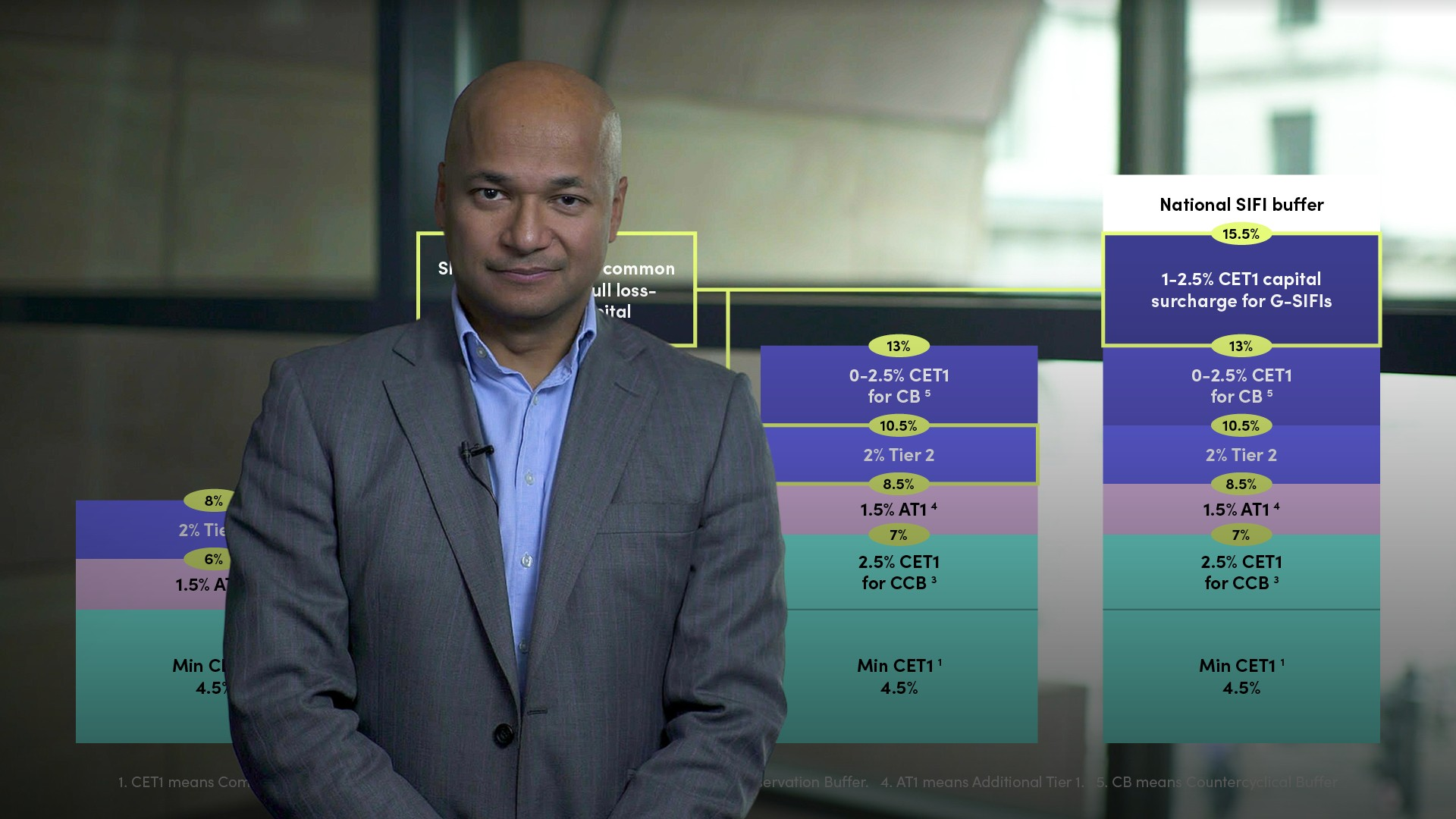11:0725:0919:44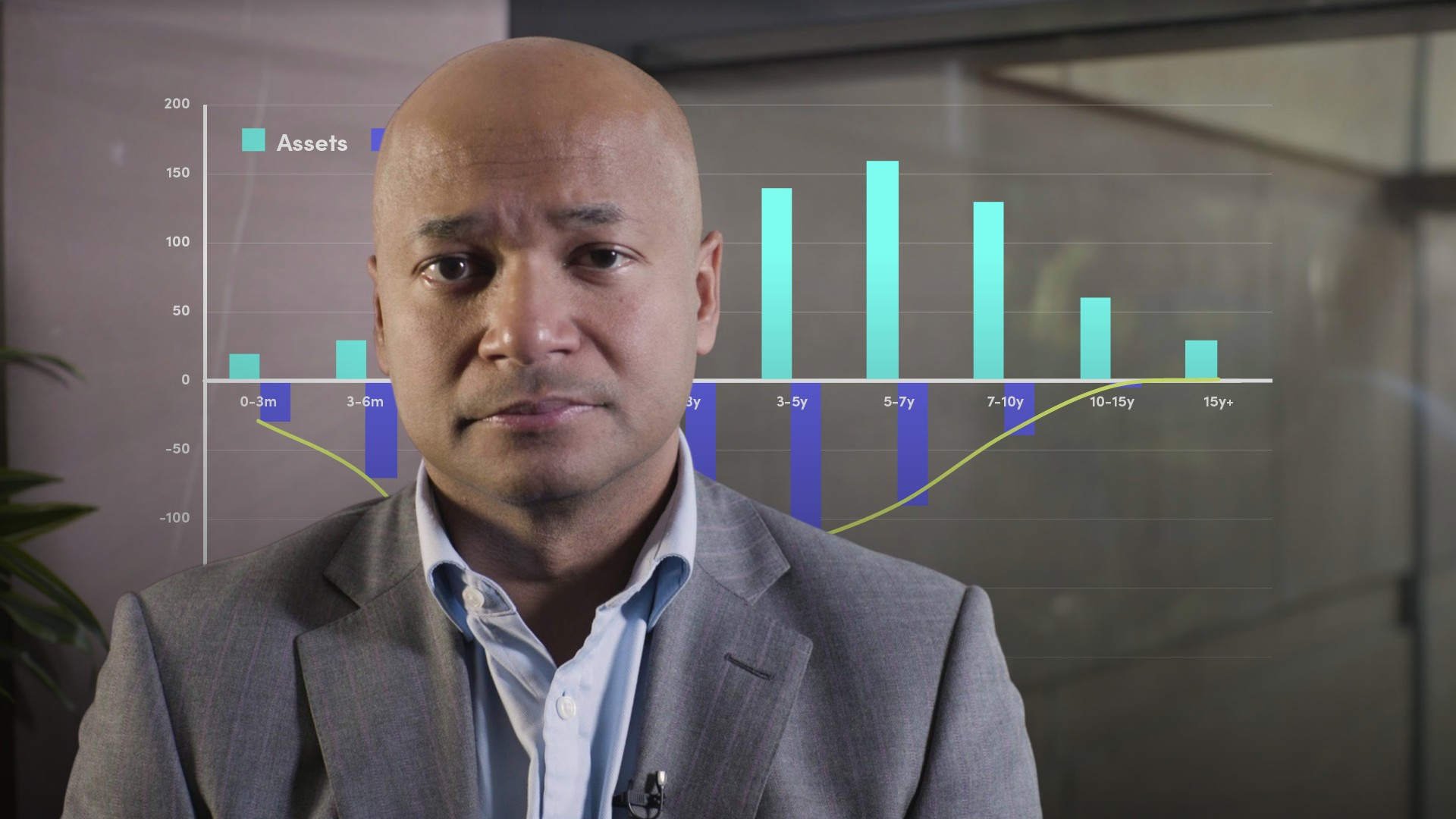17:04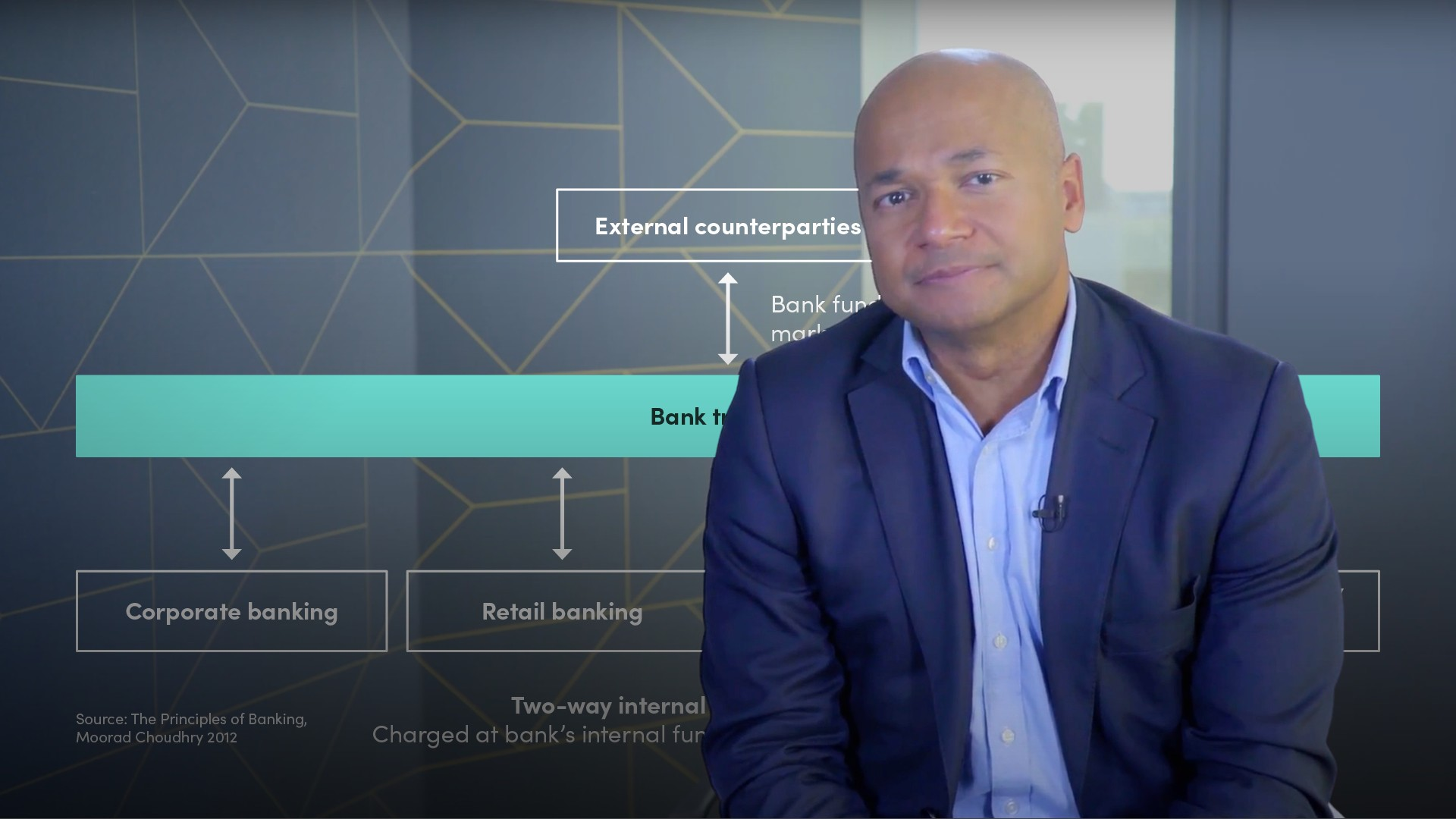16:5910:01

## Related videos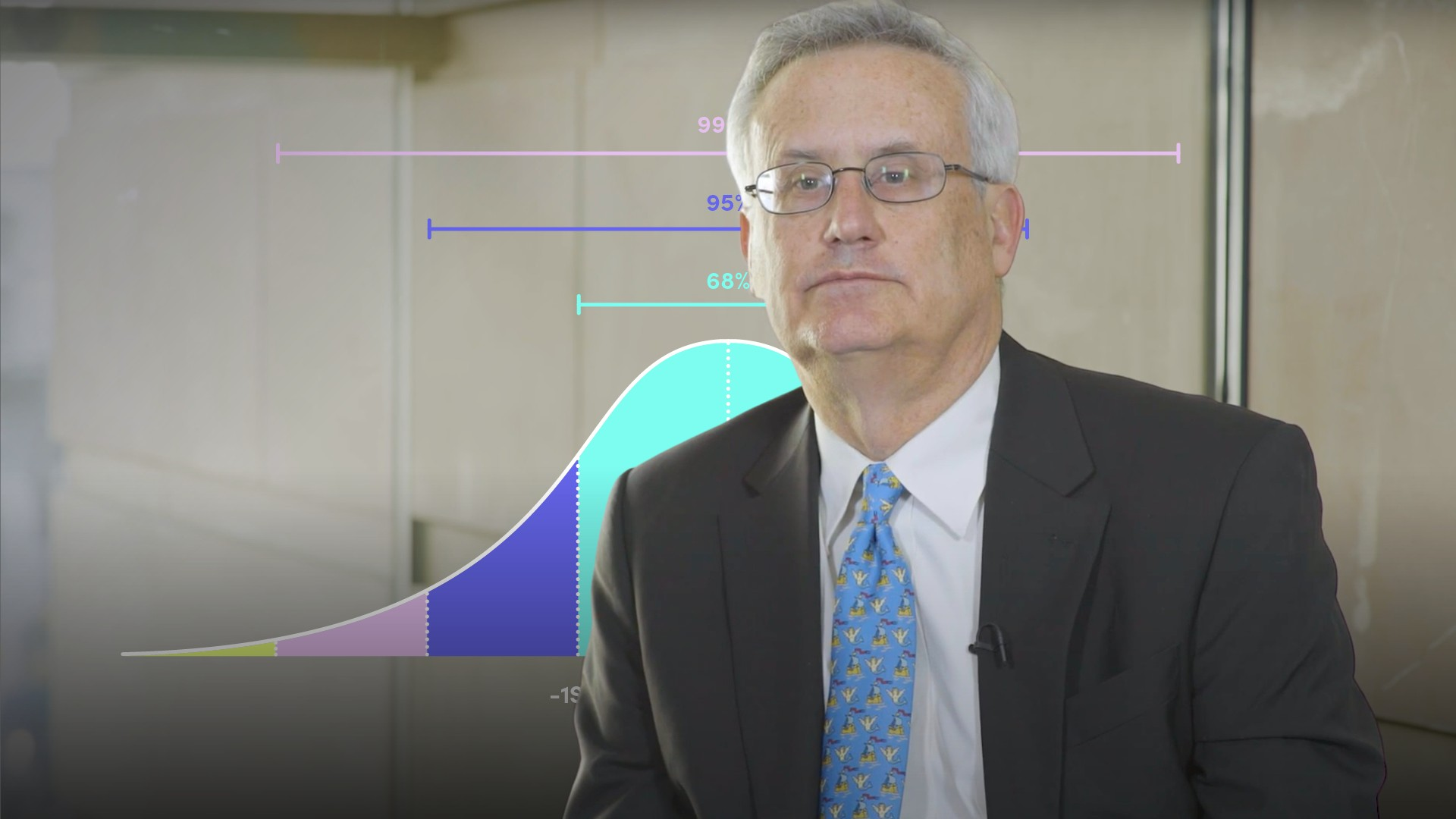Derivatives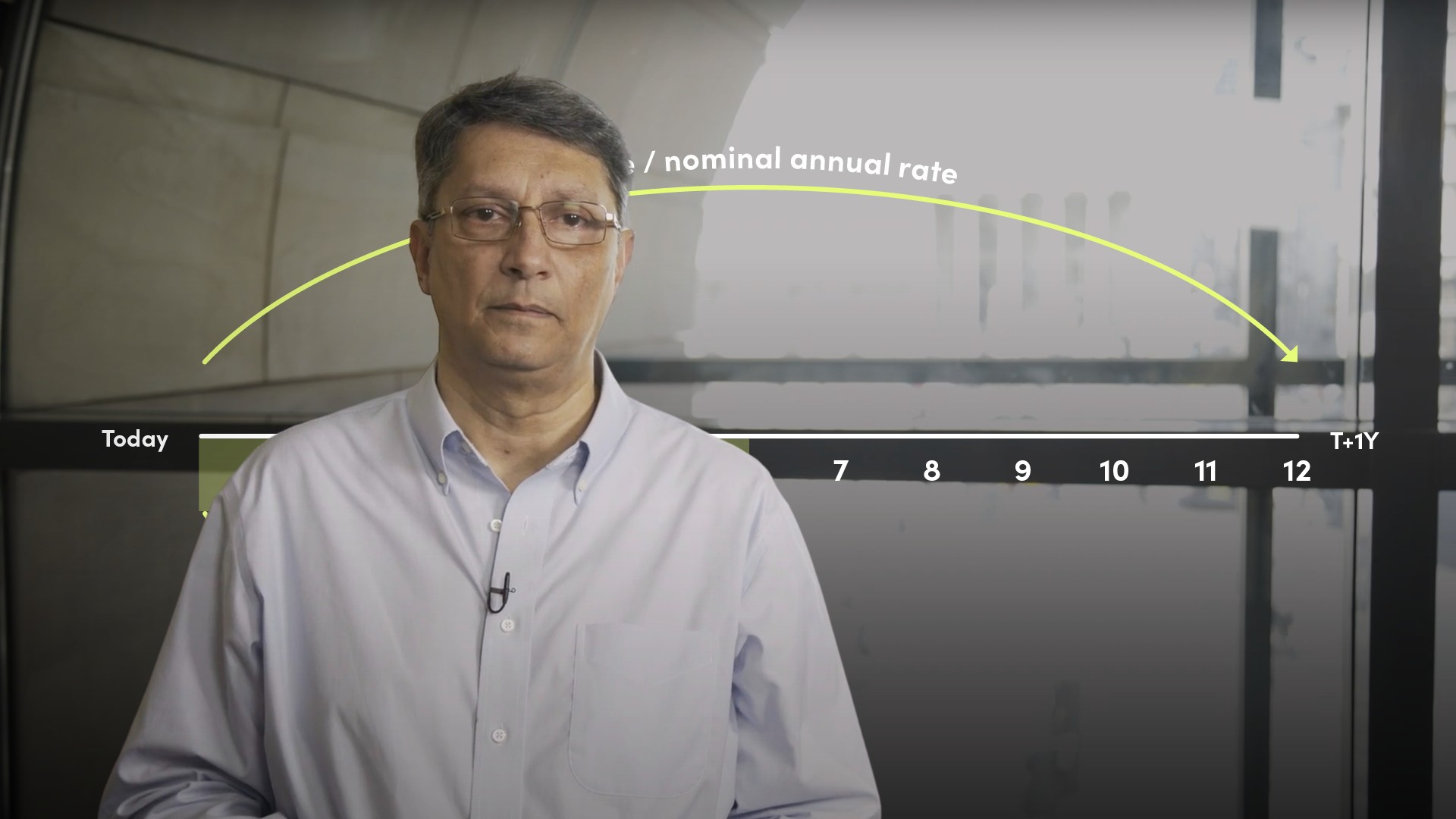Financial Maths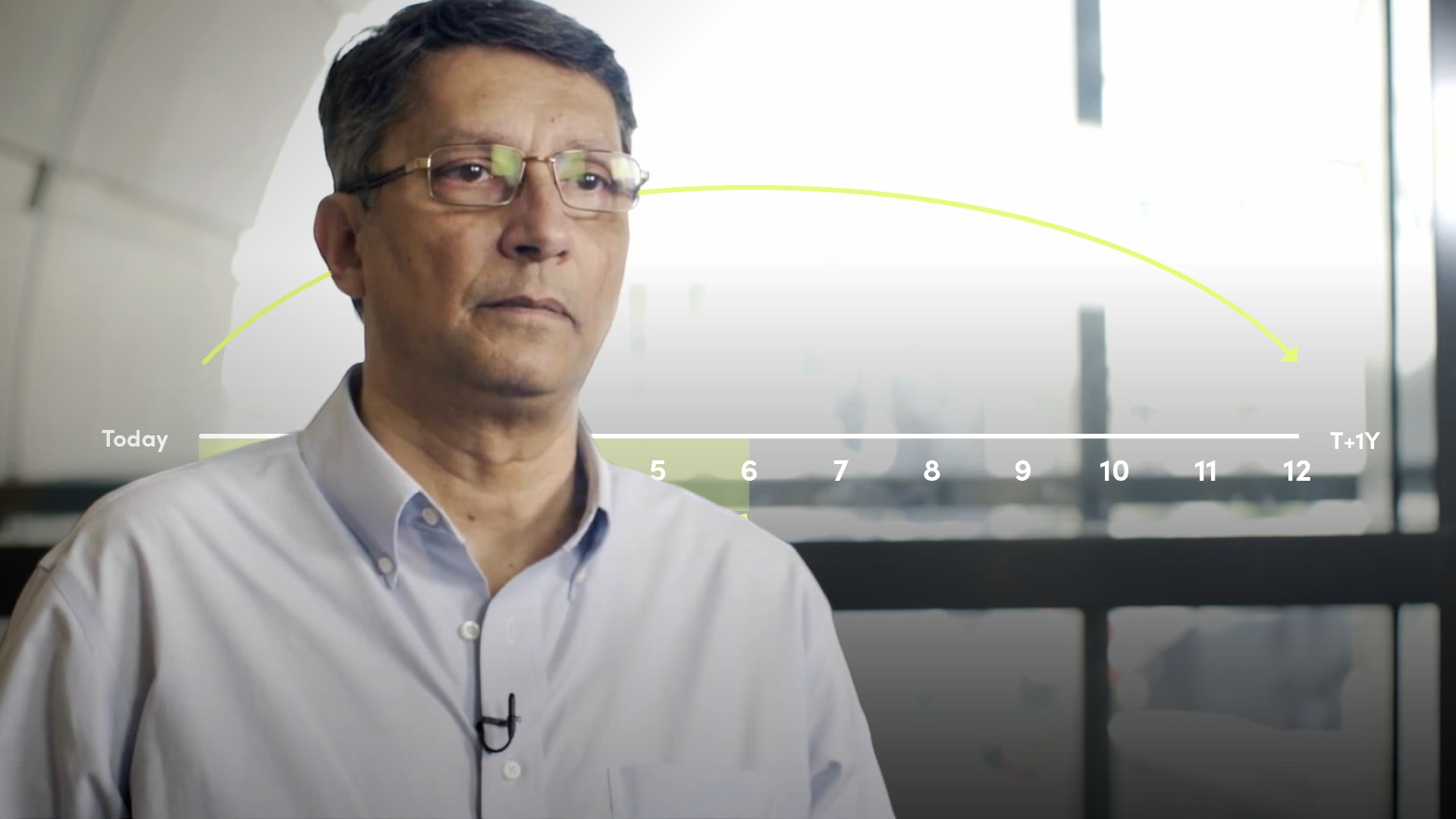Financial Maths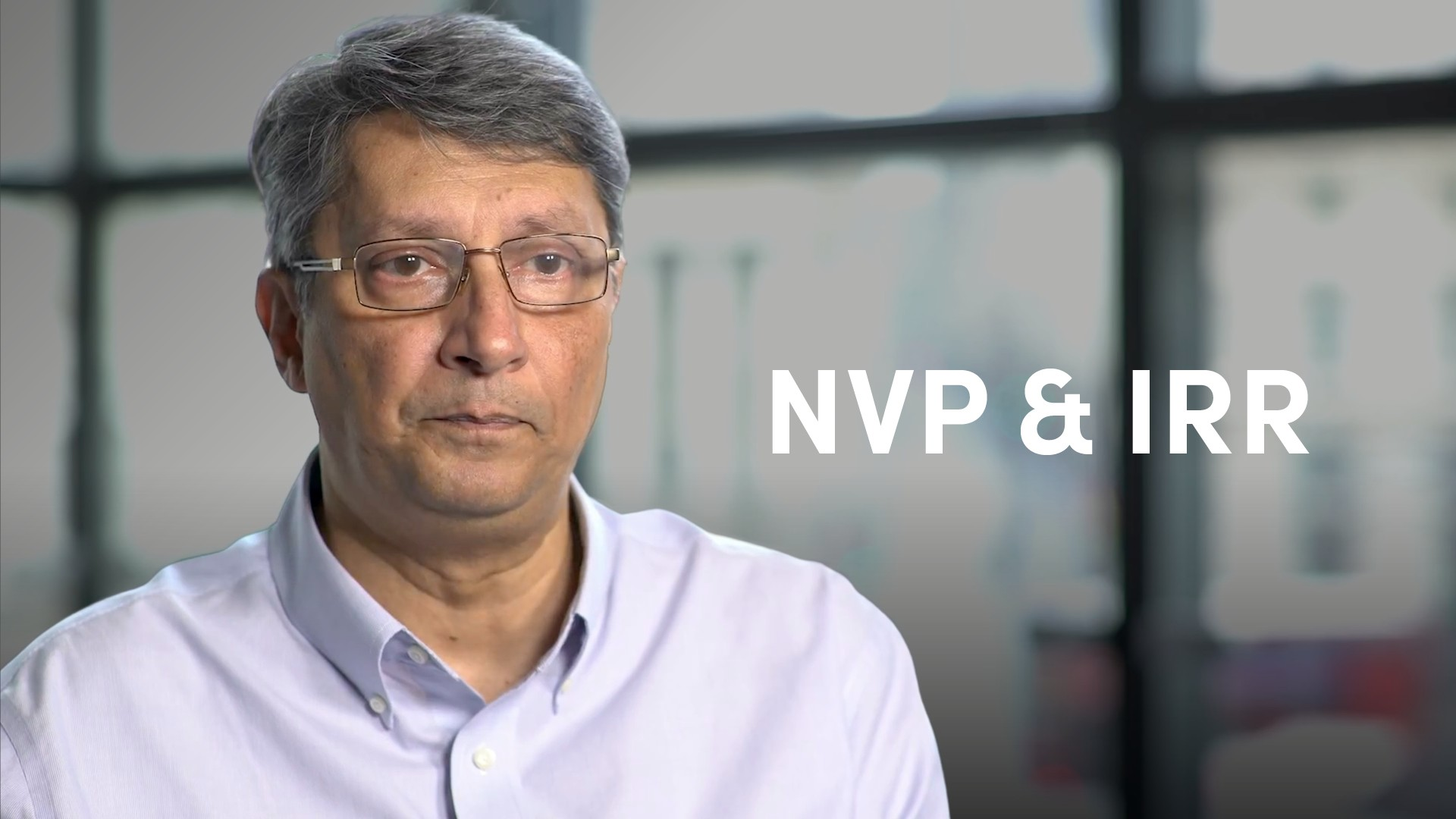Financial Maths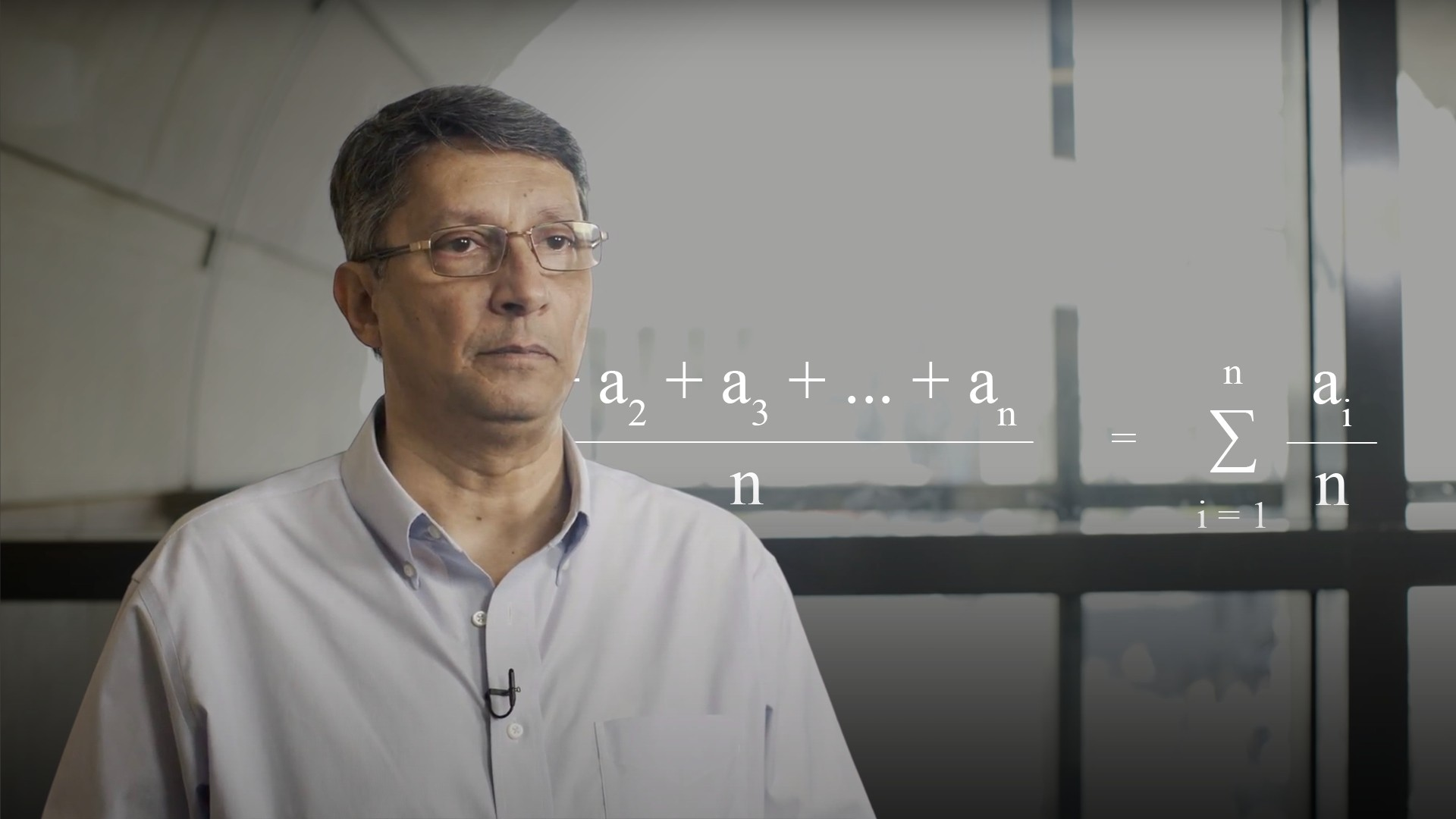Financial Maths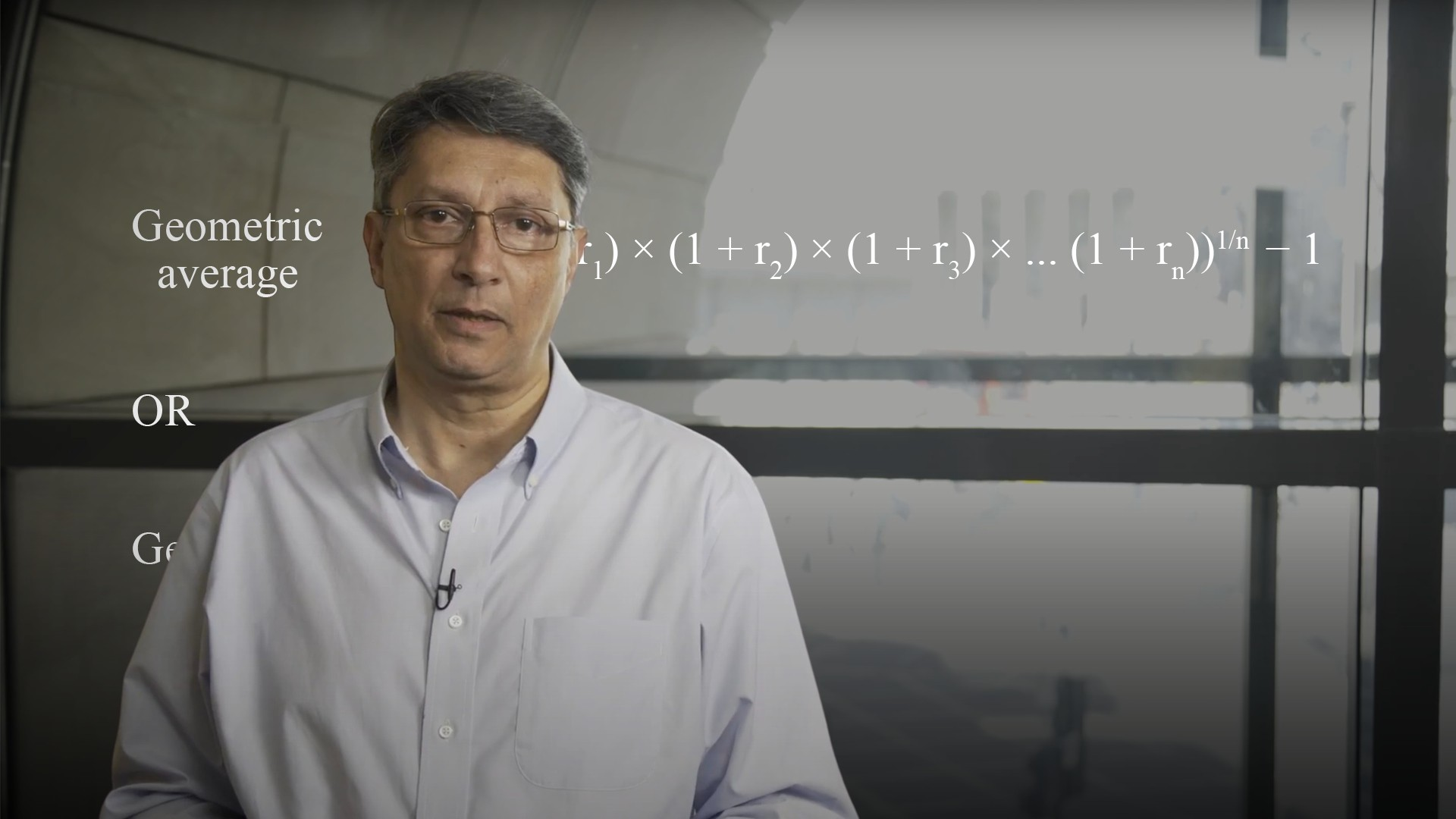Financial Maths### Accredited by